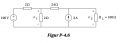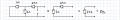# Getting a different Thevenin equivalent resistor than the answer

#### Aria Nemati

Joined Jul 19, 2019
27
Its been a while since I last worked with electric circuits.

Im trying to replace this circuit with the Thevenin equivalent.I get a Thevenin equivalent resistor of 25 ohms, the answer should be 3 ohms.

This is how I tried to solve it. What did I do wrong?#### InterestingStuff

Joined Nov 6, 2019
13

Perhaps the person calculating the solution for the book used 2ohm instead of 24ohm. Then you'd get 3ohm for Rth.

Quick tip to check Rth value using any circuit simulator: Redraw the circuit shutting down all non-controlled sources (like you did) and setup a "test" voltage or current source between points a-b (a-b being the points for the thevenin equivalent)

Measure the current (or voltage) between the two points. Vab/Iab is your Rth.

•Aria Nemati

#### Aria Nemati

Joined Jul 19, 2019
27
Thank you. I guess the solution is wrong. Could you help me out with calculating the Thevenin equivalent voltage source? The answer should be 125V. I dont know how to do it. I tried with node voltage but I dont know how to do node voltage when I have a resistor (24 ohms) and a current source (3A) in the same branch. I get (v1-100v)/24ohms + v1/2 ohms but dont know what to do with the 24 ohms resistor and the 3A current source. also tried with the mesh current method, but I havent learned to do mesh current method with a current source.

#### WBahn

Joined Mar 31, 2012
27,855
Its been a while since I last worked with electric circuits.

Im trying to replace this circuit with the Thevenin equivalent.
View attachment 192116

I get a Thevenin equivalent resistor of 25 ohms, the answer should be 3 ohms.

This is how I tried to solve it. What did I do wrong?

View attachment 192117
The only thing you did "wrong" was to assume that the supplied answer is correct.Seriously, whenever you get an answer different than what you think it should be (for whatever reason), always be willing to consider that your answer is right and the answer you thought it should be is wrong.

To do that you need to develop the ability to verify the correctness of a solution, be it the one you came up with or the only you've been given or wherever it may have come from. Then you should get in the habit of ALWAYS checking the correctness of the answer you came up with whenever it is possible. The good thing about most engineering problems is that it is almost always possible to verify the correctness of an answer.

One good way to do that is to look for limits on the possible range of answers.

In this case if we look at the circuit to the left of the 24 Ω resistor we see that the absolute lowest Thevenin resistance it can have is 0 Ω (by assuming that it is replaced by an ideal voltage source). That means that we have an ideal voltage source in series with 24 Ω, so when we transform that to a Norton circuit we would have 24 Ω in parallel with two current sources. The only approximation we made was to ignore the resistance of the left hand part of the circuit and that would only increase the equivalent resistance, so the LOWEST that the equivalent resistance can possibly be is 24 Ω. Therefore an answer of 3 Ω is not even in the ballpark.

•Aria Nemati

#### WBahn

Joined Mar 31, 2012
27,855
Thank you. I guess the solution is wrong. Could you help me out with calculating the Thevenin equivalent voltage source? The answer should be 125V. I dont know how to do it. I tried with node voltage but I dont know how to do node voltage when I have a resistor (24 ohms) and a current source (3A) in the same branch. I get (v1-100v)/24ohms + v1/2 ohms but dont know what to do with the 24 ohms resistor and the 3A current source. also tried with the mesh current method, but I havent learned to do mesh current method with a current source.
How do you get that the voltage across the 24 Ω resistor is (v1 - 100 V)? That is the voltage across the top 2 Ω resistor (measured as the right side relative to the left side).

Apply KCL at the node above v1.

BTW -- Thanks for tracking your units in your equations -- so very rare to see someone do that.

#### Aria Nemati

Joined Jul 19, 2019
27
How do you get that the voltage across the 24 Ω resistor is (v1 - 100 V)? That is the voltage across the top 2 Ω resistor (measured as the right side relative to the left side).

Apply KCL at the node above v1.

BTW -- Thanks for tracking your units in your equations -- so very rare to see someone do that.
Sorry. I dont know why I wrote 24. Silly mistake. I meant to type (v1-100V)/2 ohms.

#### WBahn

Joined Mar 31, 2012
27,855
Sorry. I dont know why I wrote 24. Silly mistake. I meant to type (v1-100V)/2 ohms.
Okay, so [(v1 - 100 V) / 2 Ω] + [v1 / 2 Ω] is the current flowing out of the node. What is the current flowing into the node?

#### Aria Nemati

Joined Jul 19, 2019
27
Okay, so [(v1 - 100 V) / 2 Ω] + [v1 / 2 Ω] is the current flowing out of the node. What is the current flowing into the node?
I see. Its 3 Amps. Which gives us a total of 53V at the node above v1. The voltage over the 24 ohms resistor is 3A*24 ohms = 72V.
We get Vth= 53V+72V = 125V. Thanks for the help. Now I can finally sleepDoes this still count as "node voltage method" ?

#### WBahn

Joined Mar 31, 2012
27,855
I see. Its 3 Amps. Which gives us a total of 53V at the node above v1. The voltage over the 24 ohms resistor is 3A*24 ohms = 72V.
We get Vth= 53V+72V = 125V. Thanks for the help. Now I can finally sleepDoes this still count as "node voltage method" ?
It is certainly related to the node voltage method, but I don't know that I would go quite so far as to call it that -- but that's a matter of semantics, really.

The node voltage method is really nothing more than a systematic way of applying KCL to the essential nodes in a circuit in such a way that the resulting system of equations ensures the satisfaction of KVL around all possible loops. In a similar vein, mesh current analysis is nothing more than a systematic way of applying KVL around an appropriate set of loops in a circuit in such a way that the resulting system of equations ensures the satisfaction of KCL at all node.

•Aria Nemati